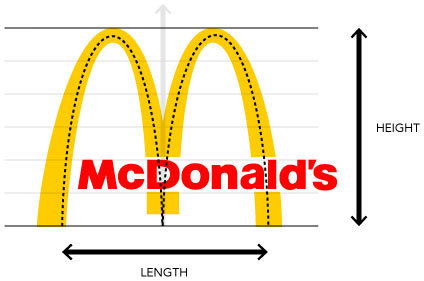# Figure Out The Mathematics Behind The Famous Golden ArchesIf the McDonald's logo were stored as a set of pixels, enlargement would quickly result in distorted or pixelated images, which are an eyesore. As such, companies often make vector images of their logos, in which the information is stored as mathematical formulae. Such vector images are easily scaled while maintaining sharp, crisp images.

As a first approximation, the logo is deconstructed and approximated as 2 parabolic curves of the form $y = -A(x-5)^2$ and $y = - A (x+5)^2$. The McDonald's logo has a height to length ratio of 1.05. What is $A$?

×